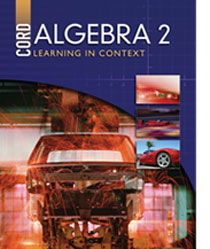# Algebra 2 - First Edition

## Chapter 3: Matrices

Some links are repeated for use with more than one lesson.

### The following links are applicable to the entire chapter.

http://www.sosmath.com/matrix/matrix.html

http://edhelper.com/Matrices.htm

### 3.1 Matrix operations

http://www.sosmath.com/matrix/matrix0/matrix0.html

### 3.2 Matrix Multiplication

http://www.purplemath.com/modules/mtrxmult.htm
This page contains a tutorial on matrix multiplication and an animation on how to use your hand to keep track of the steps.

http://www.mai.liu.se/~halun/matrix/

http://www.easycalculation.com/matrix/matrix-multiplication.php

### 3.3 Determinants

http://www.purplemath.com/modules/determs.htm